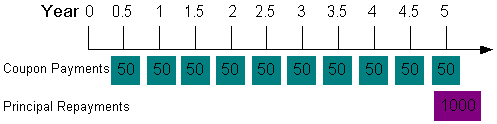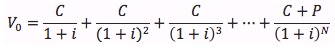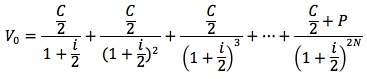### Why should I choose AnalystNotes?

AnalystNotes specializes in helping candidates pass. Period.

##### Subject 1. Bond Prices and the Time Value of Money

The idea that the value of any financial asset equals the present value of its expected cash flows is the fundamental principle of valuation. This principal is applicable to bonds, stocks and other financial assets. The present value of cash flows is calculated by discounting the cash flows at appropriate interest rates.

The cash flows of a bond have two components: periodic coupon payments and principal repayment at maturity, or when the bond is retired. Both the amount and the timing of the cash flows should be identified to value the bond.

For example a five-year Treasury coupon note with a par-value of \$1,000 has a 10% coupon. Its cash flows are as follows:For a given discount rate, the present value of a single cash flow to be received in the future is the amount of money that must be invested today to generate that future value. The present value of a cash flow will depend on when a cash flow will be received (i.e., the timing of a cash flow) and the discount rate (i.e., interest rate) used to calculate the present value.i = the discount rate
t = the number of years to receive the cash flow

The sum of the present value for a security's expected cash flows is the value of the security:N = the number of annual periods till maturity

The convention of the bond market is to quote annual interest rates that are double semi-annual rates. For most bonds coupon payments are semi-annual. Coupon payments are adjusted by dividing the annual coupon payment by two and adjusting the discount rate by dividing the annual discount rate by two:The differences are:A bond is priced at a premium above par value when the coupon rate is greater than the market discount rate. It is priced at a discount below par value when the coupon rate is less than the market discount rate. The amount of any premium or discount is the present value of the "excess" or "deficiency" in the coupon payments relative to the yield-to-maturity.

Yield-to-Maturity is the interest rate that will make the present value of the cash flows from a bond equal to its price. It is the promised rate of return on a bond if an investor buys and holds the bond to its maturity date.

Consider a 10%, two-year bond selling for \$1,036.30 (selling at premium). The cash flows for this bond are (1) four payments of \$50 every six months, and (2) a payment of \$1,000 two years from now.

\$1036.30 = \$50/(1 + YTM/2)1 + \$50/(1 + YTM/2)2 + \$50/(1 + YTM/2)3 + \$1050/(1 + YTM/2)4. Through trial and error or by using a financial calculator, YTM is found to be 8%.

User Comment
hanny asset's value = pv of its expected CF
jaimaa good and simple
youyoumm Can anyone show me how to use Texas calculater to compute the sample of YTM step by step? Many thanks!!!
yekky Sure youyoumm. Take the first example for the YTM where it mentions a 10%, 2 year bond, selling at 1036.30. Also, I have gone into some simple detail to explain this in case anyone else is having difficulty.

Very simply, always think of these bond style calculations as this: You are always given at least 3 of the inputs, if not 4 of them and you have to find one of them. Its like basic algebra where you have to solve for x.

Here the YTM or I/Y on your calculator is the variable we need to find, or our x.

Also, make sure you realize that the data in this question is given to you on an annual basis and you need to find it semi annually (like for most Level 1 questions).

So take note of all the data available, to input into your Texas BAii plus calculator:

N = 4 (annually its 2 years, so thats 2 coupon payments. But we want the semi-annual data so thats twice every year OR 4 coupon payments. Try to think of N as the number of coupon payments rather than time)

I/Y = x (we need to SOLVE for this)

PV = -1036.30 as given to us which is the premium price we paid for the bond. NOTE: Make sure you put the negative sign in front of the PV value or else your calculator will give you an error. We do this simply because the only time we are GIVING CASH AWAY is when we buy the bond right? We bought it at 1036.30 so its a negative sign to show that we have spent that money to buy the bond.)

PMT = 50(PMT = payment we receive from the coupons which is again on a semi-annual basis. So the question tells us the bond is a 10% bond meaning it pays 10% interest to us annually. But we want the semi-annual data so instead of 10% yearly, we are receiving it every 6 months so ist 10/2 = 5%. All you do now is find out what 5% of 1000 is so that you know how much interest payment we receive every 6 months = \$50.)

FV = is the principal payment we receive at the end of the bonds maturity. So its obviously going to be the \$1000 as we always regard the principal or par amount to be \$1000.

So now that you know how each input is found, you have all your values to input. You can do this simply by starting from the N and working your way all the way to the FV or however you like to do it. Note: Remember that on the Texas Baii plus, you enter the values with the number first. So for example if you N = 4, you press 4 first and then N and thats how you plug in all the other data as well.

So type in the data like this:

4 = N

(skip I/Y as thats what we need to compute at the end)

-1036.30 = PV

50 = PMT

1000 = FV

then press the yellow 2nd button and then press CPT (compute) and then press the I/Y button.

= it should give you an answer of 4.

Remember you just solved everything on a SEMI-ANNUAL BASIS. So if the SEMI value is 4, the WHOLE value is your 4 multipled by 2 = 8% ANNUALLY.

I hope you actually made it to this point because such calculations are crucial for the exam. All the best.
2014 Yes best fast
gill15 I finished all these sections and there is a TON of people who have no idea whats going on. The actual textbook notes are not that long. If you know what you're doing this section is easy. Just read the textbook. Some of the comments and questions for bonds are just beyond believable.
vatsal92 DCF is just the PV of all expected future cash flows from a project.
CFAToad Anyone interested in learning the HP12C should start on YouTube. Just type in "HP12C [subject]" into Google. Then click on videos.
Inaganti6 yekky you're a diamond of a human being
blackdeath Can Someone explain how the given formula is derived and what is the basic principle behind it with a simple example?
edrei7 There is no complex derivation of the formula. You are just discounting your future cash flows (interest and principal) to the present day using a certain discount rate (market-prevailing rate).
edrei7 The YTM is just the IRR equating the bond price and the discounted cash flows of interest and principal.
anandstudy Use cashflow function:
CF0 = -1036.30
C01= 50
F01= 3
C02= 1050
IRR =CPT = 4.00
Because this is a half yearly rate, it should be multiplied by 2 to get the annual rate of 8%